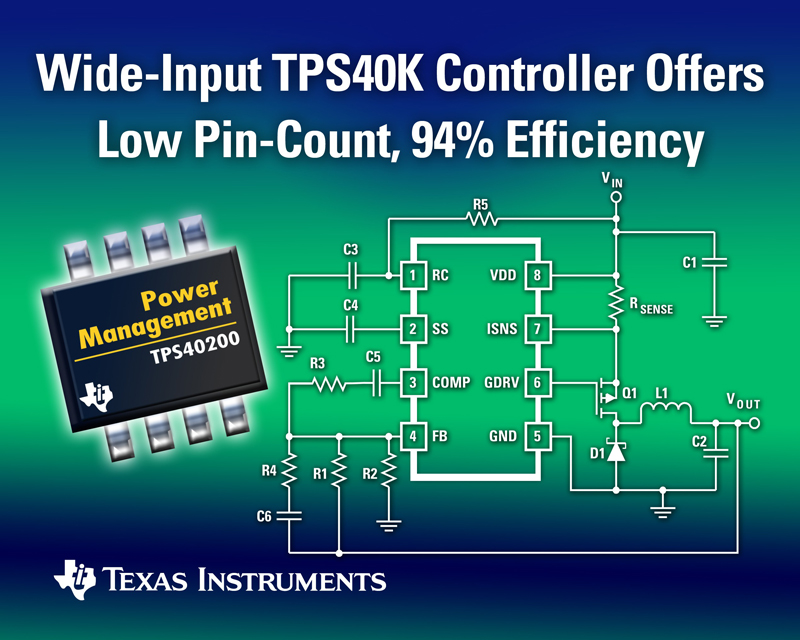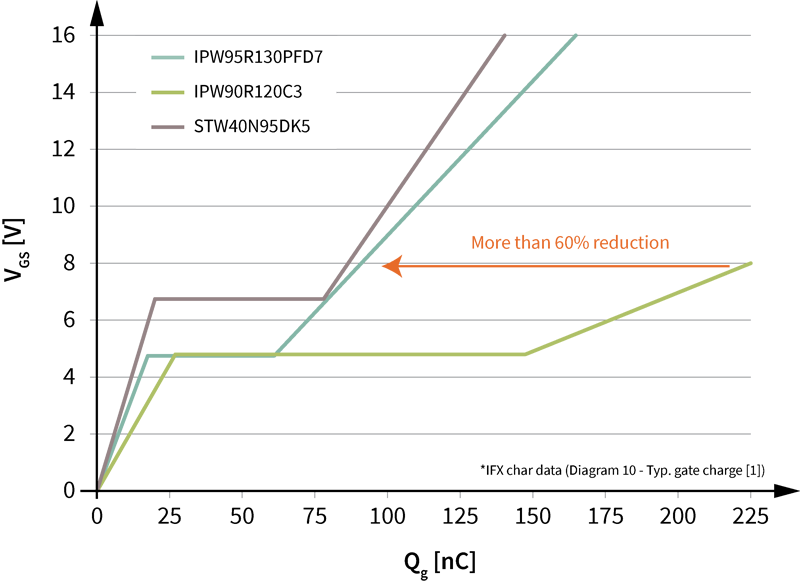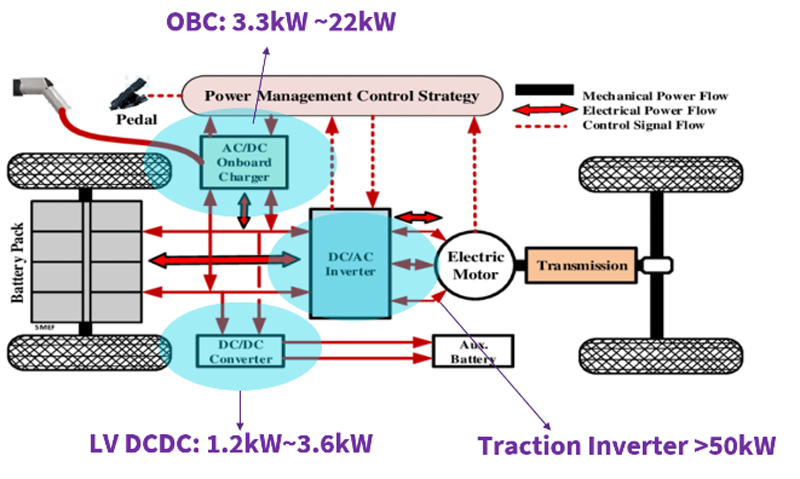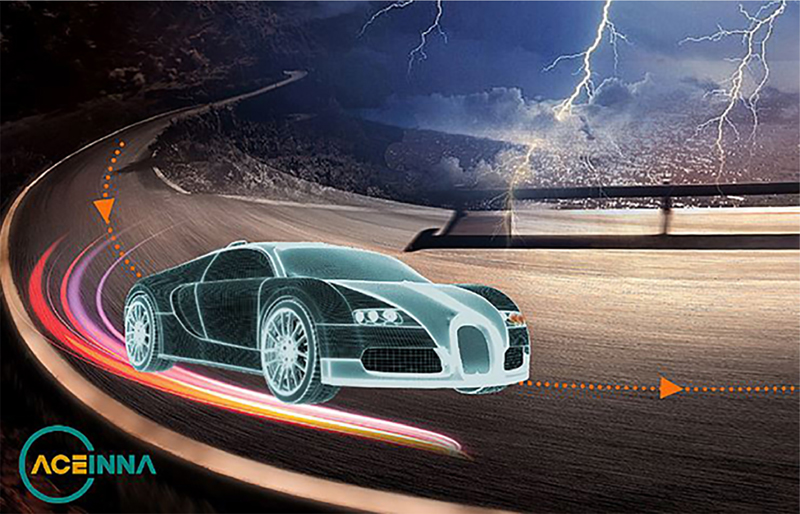# High Power solutions

Author:
Vincenzo Pizzolante, Texas Instruments

Date
02/03/2012PDF
##### Using TI controllers

Many applications in power management are based on buck topology. The purpose of this application note is to describe a technique to overcome the current limitation of these solutions. The technique is perfectly supported by TI buck controllers. In industrial environments, a typical power management application is to obtain all the lower voltage rails from a (shared) power bus (typ. 12, 24 or 48V). A common limitation for all the available controllers is the power rating: the maximum current for a single phase solution is 30Amp. The cause of this limitation is the inductor. In a buck converter, inductor selection is driven by peak-to-peak inductor current ripple. As rule of thumbs, it is set around 20% of maximum output current. It is well known that to reduce the previous ripple, the designer can act on the value of inductance L and / or the switching frequency FSW (input and output voltages are supposed to be assigned). FSW is chosen in order to maximize efficiency: again, as rule of thumb, the selection of this value in driven in order to have: Switching Losses ? Conduction Losses since this would guarantee the best performance (in terms of efficiency and then thermal stability) of the system. This means that, in other words, the equation that rules the ripple current is used to select the value of L. High values of L lead to high values of DCR that, as well, lead to high temperatures: since any component or part has a maximum temperature rating, the limitation in maximum current that can flow across the inductor is well understood (from the moment in which high L should be preferred to keep the current ripple under control, while low L should be selected to keep losses and inductor temperature under control). On the other side, low DCR are obtained for low L or, in other words, that to keep the ripple under control the design has to act on FSW, violating the condition for the maximum performance. Another limitation in using high L values is that the dynamic response of the system would be poor, meaning a big (and expensive) amount of output cap should be used in order to contain the output voltage ripple within the required window (in particular when load transient events occur). Solutions To override the previous limitation one of the following solutions can be adopted:

• To use the same controller / solution and increase the amount of power available at the output paralleling the "power components"
• To move on a multiphase architecture
• To adopt an hybrid solution, as proposed in this paper
Paralleling components gives poor efficiency but the original solution / controller doesn't need to change. Indeed, a controller with embedded driver like TPS40200 had to switch many power FETs in parallel and because the limited peak current, a limitation in number of components that can be paralleled is defined. The switching speed of the FETs will result even poor and this normally reduces efficiency. Multiphase controllers offer better performance and features than single phase with parallel parts, but are more expensive as well: high pin-count, many components needed for settings, high cost of the whole solution. A new third approach is presented in this paper: to keep the power structure of the VRMs and replace the multiphase controller (bigger and expensive) with a single phase controller. This is useful in all the applications that, for their own power rating, rely in the "gray zone" for which a single phase solution starts to be "borderline" and the minimum multiphase solution should be adopted (two phases). The TI TPS40200 controller has been considered for the purpose, even if the results can be extended to the other PWM controllers. Implementation The idea is to use a single high frequency PWM signal and split it in two phase shifted signals, by using a frequency divider. A high frequency PWM signal drives a D-Type Flip-Flop connected in T-mode. This means at each PWM pulse the signal Q turns its polarity, allowing PWM1 = PWM or PWM1 = 0 (similar comments apply to /Q). In this way, the result is that input signal pulse train is split: the odd pulses form PWM1, while the even ones form PWM2. Making a OR gate of PWM1 and PWM2 the initial PWM (time shifted) is obtained again. In order to avoid skew issues between the signals entering the AND gates, the PWM signal is clocked on the falling edge: indeed, without the NOT gate the PMW rising edge reaches the AND gates before Q1 and Q2 have changed their polarity; this could result in glitch and short "false pulses" on PWM1/2 side. The previous cell can be cascaded: a 4 phases system can then be obtained (in general 2^N phases, in which N is the number of the PWM splitter cells) from a single PWM generator.Dual Phase Solution Based On Ti Tps40200 All the pins of TPS40200 keep their native function: RC (pin 1) for the FSW programming, SS (pin 2) for the soft start programming, COMP & FB (pin 3, 4) to stabilize the system, GDRV (pin 6) is the high frequency PWM output, ISNS (pin 7) for the overcurrent protection. GDRV output is supposed to drive a PMOS, meaning it must be inverted in polarity and re-scaled in order to meet the logic levels of the frequency divider illustrated above. To implement the over current protection a hybrid approach is adopted: it consists in using the DCR of the input LC filter (always present in buck solutions). Indeed, it is possible to demonstrate that if the Time Constant Matching condition is satisfied then the voltage between pin 8 and pin 7 of the controller is equals to: LIN / DCR = R x C [time constant matching condition] VDD - VISNS = DCR x IIN = VILIM [overcurrent condition] In which: LIN, DCR are assigned by design; VILIM is provided in TPS40200 electrical characteristics; IIN is the current that flows into LIN (the input filter choke); R and C are chosen in order to meet the time constant matching condition; In this case the OCT level is set by the choice of the inductor: if it shouldn't be possible to get an inductor with the right DCR, than an extra resistor R' can be put in parallel to the time constant matching capacitor. In this case: LIN / DCR = R' // R x C [time constant matching condition] VDD - VISNS = R' x DCR x IIN / ( R' + R ) = VILIM [overcurrent condition] Now R, R' and C are chosen in order to allow the system to trigger an over current event at the desired value of IIN. Since less values for C are available it is set arbitrarily (typ. 10 or 100nF), while R and R' are calculated solving the previous 2x2 system of equations. Conclusions In this application note a cost and space efficient controller is turned into a powerful VRM. The results are general and can be reused with many other TI devices as well. More features typical of VRM can be added in order to improve efficiency at light load, like the dynamic phase shedding. www.ti.com

### RELATED#### Ultrahigh power density solutions for energy-efficient high-power lighting and industrial SMPS applications

Jan 23,2023#### Wolfspeed Enables Higher-Voltage Batteries for EV Systems

Jan 4,2023#### Power Modules Accelerate the Path to Long-Haul, Autonomous Trucking

Dec 31,2022Jan 1,2023